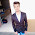# Dc Motor Speed Control

### PWM Speed Controller Using Arduino Uno

In this post, I will tell you how to make Motor speed Controller using PWM Signal.

Step - 1
Make The Circuit According To the fig -Dc Motor speed control

Step - 2
Collect the Following Components -
• Arduino Uno
• L293D IC
• 47 Kohm Potentiometer
• Slide Switch - 2
• 9 Volt Battery
• Dc Motor
• Some Wires

Step - 3

Make All Connections According to the fig -

Battery +             ------->  VIN
Battery -              ------->  GND
Regulator Left pin ------->  GND
Regulator Middle pin ---->  A0
Regulator Right pin ------> 5 Volt
IC Pin 1                 ------> 7
IC Pin 2                 ------> 6
IC Pin 3                 ------> Motor Pin one
IC Pin 4 & 5           ------> GND
IC Pin 6                 ------> Motor Pin Two
IC Pin 7                 ------> 5
IC Pin 8 & 16         ------> VIN

Step - 4
Upload this Code In Arduino Uno
``````
/* You Can Also see the Output detail In Serial Moniter */

#define speed_1     7          /* Ic Pin Number 1 Speed Control pin */
#define left_1      6          /* Connect To Motor */
#define left_2      5         /* Connect To Motor */
#define left_reg_1  A0         /* Regulator Middle Pin Connect Here */
#define forward_1   11         /* Foward Switch Connect Here */
#define backward_1  12         /* Backward Switch Connect Here */
double reg_1=0.0;
double Time = 50.0;            /* You can Adjust This time For run yout Motor Smoothly range between 1 - 200 */

/* You Can Also see the Output detail In Arduino Serial Moniter */

/*
* Motor Speed Control Using PWM Signal
* Mail me on eccircuit@gmail.com
* Code Write By Ankit Jat
*/

void setup() {
Serial.begin(9600);
pinMode(left_reg_1 , INPUT);
pinMode(forward_1 , INPUT_PULLUP);
pinMode(backward_1 , INPUT_PULLUP);
pinMode(speed_1 , OUTPUT);
pinMode(left_1 , OUTPUT);
pinMode(left_2 , OUTPUT);
Serial.println("\n\t\t Wellcome \n\t Moter Speed Controller");
}

void loop() {
Serial.print("Forward \t");
Serial.print("Value of Left Regulator : ");
Serial.print(reg_1);
reg_1=((reg_1*Time)/1023.0);
digitalWrite(left_2 , LOW);
digitalWrite(left_1 , HIGH);
Serial.print(" Speed of Left motor : ");
Serial.print(reg_1);
digitalWrite(speed_1,HIGH);
delay(reg_1);
digitalWrite(speed_1,LOW);
delay(Time-reg_1);
Serial.println();
}
Serial.print("Backward \t");
Serial.print("Value of Left Regulator : ");
Serial.print(reg_1);
reg_1=((reg_1*Time)/1023.0);
digitalWrite(left_1 , LOW);
digitalWrite(left_2 , HIGH);
Serial.print(" Speed of Left motor : ");
Serial.print(reg_1);
digitalWrite(speed_1,HIGH);
delay(reg_1);
digitalWrite(speed_1,LOW);
delay(Time-reg_1);
Serial.println();
}
}
``````

Step - 5

NOTE - You can change the value of time-variable in the Code to change the PWM Wavelength.

Minimum Value of Time is 1.

You can also able to change the pin number of components in the code.
but you can change regulator Pin A0 with A0 to A6.

If You Have any question or suggestion please comment below.

Thank You

1.1.